## COLOURING THE PLATONIC SOLIDS

 Many years ago I set the following puzzle in Hot Key: In how many ways can you colour the faces of a cube using 6 colours? The colourings must be such that each coloured cube must be unique whatever way it is orientated. The answer is 30. This is the way I did it. I notionally numbered the colours 1 to 6 and chose number one as a vertical face facing me. This could always be the case. Then I asked myself 'where could I place colour number 2?' It could be adjacent to the face coloured 1 or it could be opposite. Taking the adjacent case first, the cube could be orientated by rotation so that the face coloured 2 was on top leaving face 1 facing me. This fixes the orientation and there are 4 faces left to colour so the number of colouring must be 4! (factorial 4 = 24). Considering the case when the face coloured 2 is opposite, the cube is not fixed in orientation as it can rotate without changing the assumptions so we can colour one of the other faces with colour number 3 and rotate the cube so that this face is uppermost. This will fix the orientation, leaving three faces to colour. The permutations this time are 3! = 6. These 6 are independent of the 24 we have already found so the answer is 6 + 24 = 30. If F = the number of faces; E = the number of edges; V = the number of vertices (corners), the full set of five regular Platonic solids are the Tetrahedron (F=4; E=6; V=4) Octahedron (F=8;E=12; V=6) Cube (F=6; E=12; V=8) Icosahedron (F=20; E=30; V=12) Dodecahedron (F=12; E=30; V=20) Note that in every case, V+F=E+2. This is known as Euler's theorem. The number of edges that bound each face is P=2E/F. My first question this month is very easy. How many ways can the tetrahedron faces be coloured using four colours? Same rules as before. My next question is about the octahedron which some people have difficulty in visualising. Think of it like this: place four equilateral triangles side by side so that they form a square pyramid. Now create a second square pyramid in your mind and glue their bases together. You now have an octahedron with eight triangular faces. The octahedron is the dual of the cube which means that the vertices are replaced by faces and vice versa. So you could, instead, colour the corners of the cube rather than the faces of the octahedron. (They have to be the same answer!) So, in how many ways can you colour the faces of an octahedron with eight different colours, making sure that no two colourings are the same however orientated? That's two questions in one month! New solvers need only answer the first easy one about the tetrahedron: regular solvers must do both. I hope that the puzzle this month will get you all thinking about platonic solids and permutations but if not, you may have found the puzzle page informative if nothing else. Please send your answers to me, David Broughton, to arrive by 6th August 2008.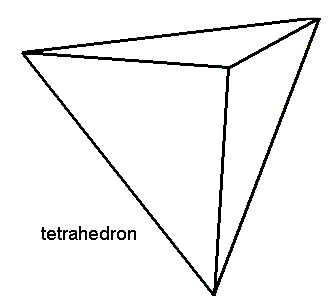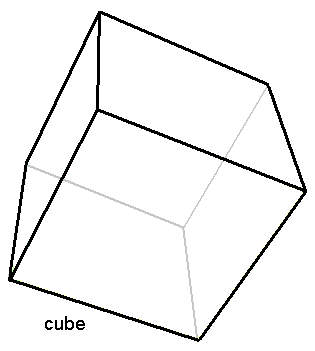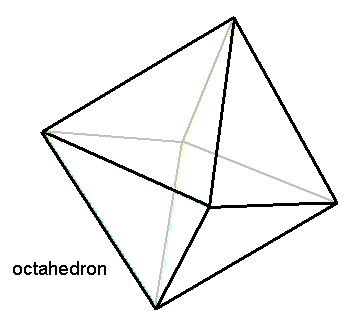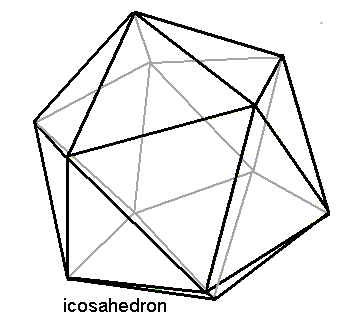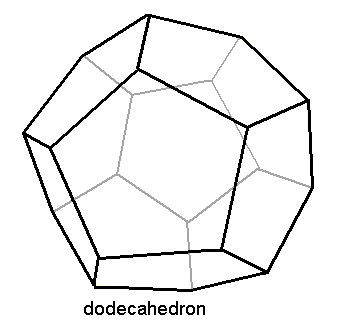If you want to colour the other platonic solids, have a go, but only for fun. Answers will be given to them all after the closing date (see below).

Click this symbol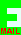for my email address.
 ANSWERS Scroll down to see the answers.                       ANSWERS There is a simple formula that I have discovered that answers this puzzle: the number of different ways you can colour a platonic solid with the number of colours equal to the number of faces is F!/2E. The following are the values of F!/2E and hence the answers to this month's puzzle. See above for the values of F and E. Those of you who are inclined that way may like to prove the formula. Tetrahedron: 2 Octohedron: 1680 Cube: 30 Icosahedron: 40,548,366,802,944,000 Dodecahedron: 7,983,360

[home] [back]